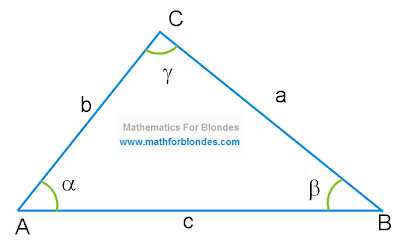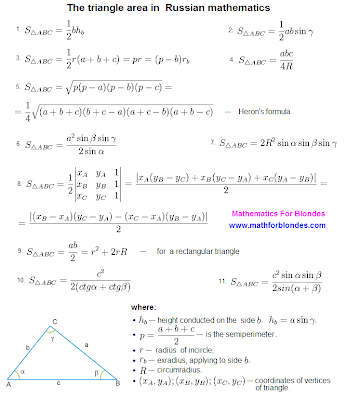1.30.2011

Triangle

The triangle is the elementary polygon. Such definition of a triangle is given by Wikipedia. We will not argue with collective reason, we will try to judge by own strength. The triangle is a geometrical figure which consists of three tops and three parties. As appears from the name "triangle", this geometrical figure has three corners. How the triangle looks? Here a triangle photo in all its beauty.Triangle

Despite such simple-minded appearance of a triangle, I very much doubt correctness of the statement of Wikipedia that the triangle is the elementary polygon. It is too much at a triangle of any gadgets. Has specially looked at the mathematical directory and here acknowledgement of my words: to a triangle it is devoted 6 (six!) the pages, to all quadrangles together taken, only 5 (five) pages. External simplicity of a triangle at all does not mean simplicity mathematical.

And so, we will start to assort a triangle on stones. Three points which are not lying on one straight line, form a triangle. These points are called as triangle tops. The request not to confuse to mountain tops is absolutely another. The triangle has three tops which are designated by the big Latin letters A, B, C (it is a surname at tops such). You ask, than these Latin letters differ from Russian letters And, In, With? A family tree at these letters different, and consequently also an order of an arrangement of these letters in the alphabet.

Between triangle tops there are triangle parties. These are such equal paths on which it is possible to run across from one top of a triangle to other top. The triangle parties also are inviolable frontiers of a triangle. In these borders there is a triangle area. Everything that is outside behind these borders, into the triangle area does not enter. Along these borders frontier guards with dogs go and watch, that another's area has not got into the triangle area, and the triangle area has not run away abroad from such good life in a triangle. The triangle parties by small Latin letters a, b, c are designated.

Just the same small Latin letters a, b, c the length of these parties of a triangle is designated. The strict boundary heads after all need to know, how many kilometres poor boundary dogs have run? How many kilometres passed unfortunate frontier guards are, for some reason, chiefs never interests. When speech comes about length of the parties of a triangle, probably other designation - two big Latin letters with two vertical sticks: |AB |, |BC |, |AC |. In this case the designation of length of the party of a triangle undertakes on a surname of tops of a triangle between which there is this party (look a photo triangle). In full conformity with rules of mathematical bureaucracy it is possible to write down:

a = |BC|

b = |AC|

c = |AB|

From here the first law of a triangle for blondes is very easily deduced: the length of the party of a triangle is designated by two big Latin буковками or one small буковкой, that which is not present among big буковок.

It is very logical to assume that triangle corners also have the designations. Each corner of a triangle has cosy settled down in triangle top between two parties. Designate triangle corners small letters of the Greek alphabet α (alpha), β (beta), γ (gamma). The sum of all corners of a triangle is equal 180 degrees. Such to itself democracy of a triangle: if you a corner also want to be more, select at other corner and use. All as in life. Therefore corners in a triangle meet the different: stupid and pompous (you such perfectly know) near to thin and graceful (blondes). Democracy of a triangle in the mathematician is called "the triangle Theorem" and it sounds so: the sum of all corners of a triangle equals hundred eighty degrees. In mathematical symbols the triangle theorem looks so:

α + β + γ = 180°

Depending on a kind of the corners which have formed Open Company "Triangle Ltd.", triangles differ on appearance. It is such geometrical dress-code for triangles. But about it we will talk next time.

Here you will find answers for such questions: the sum of all corners on a triangle.

1.29.2011

The triangle area

The triangle area concerns that number of school problems which very often should be solved and in the further life. Now we speak not about the area of a triangle Bermudas which at desire can be calculated (by the way, it makes more than one million square kilometres), and not about the area of a triangle love which basically it is impossible to calculate. We speak about the area of that triangle which is a geometrical figure. By the way, from a figure of the blonde of mathematics something took for the favourite triangles. But about it another time.

Today we will look one eye at formulas of a triangle for an area finding. The triangle area in Wikipedia is given in the form of the whole heap of formulas. I стырил them therefrom also have made in the most unscrupulous image for you a crib on geometry with formulas of the area of a triangle and a portrait of the hero of the festivities - a triangle. This crib on favourite our mathematics can be downloaded free of charge - the right button of a mouse (fiiii!) "to keep drawing as". This crib remains at you in the computer.The triangle area
Let's begin with a triangle picture. In different textbooks there can be different designations of tops, the parties and triangle corners. Therefore, before it is stupid to apply formulas from a crib to the decision of the problems, compare all designations of the parties, corners and tops of triangles. Quite probably that some letters in formulas to you should be changed, what on a crib and in your textbook there were identical designations.

Now about formulas. Under figure 1 (one) costs the most widespread formula for a finding of the area of a triangle in length of the party of a triangle and the height of a triangle lowered on this party. The area of a triangle to half of product of the party of a triangle on height equals.

The second formula allows to find the triangle area on length of two parties and a corner between them. At the formula there is a sine of the angle the scale for which value it is necessary to search under the trigonometrical table or to calculate on the calculator.

The third formula allows to find the triangle area on three parties and radius of the entered circle. Equal-signs in this formula divide different variants of this formula in which the triangle semiperimeter, radius of the extraentered circle, concerning is applied by one of the parties. With these features we somehow will make an effort understand. For now below our crib it is possible to find that designation who is who in our triangular zoo. And formulas for calculation of height and triangle semiperimeter.

On lengths of three parties and radius of the described circle it is possible to find the area by means of the fourth formula.

My most favourite formula - the formula of Geron - at number five. This formula allows to find the triangle area on three parties. Than so this Heron's formula is good? On work I with its help calculated the area of almost any geometrical figure. For example, if the room had the form of a wrong polygon was to measure lengths of walls and distance between room corners (lengths of the parties and distance between polygon tops enough). Then under the formula of Gerona the area of triangles into which it is possible to break any polygon was calculated. The sum of the areas of triangles gave the polygon area, that is the room area. In a crib the Heron's formula is presented in two variants - a finding of the area of a triangle through semiperimeter and in lengths of the parties.

Further we can find the triangle area on one party and three corners (the formula 6), on radius of the described circle and three corners (the formula 7), on co-ordinates of tops of a triangle (the formula 8). In last formula vertical sticks in numerator designate the number module, after all the area cannot be negative are mathematicians know even.

The area of a rectangular triangle (the formula 9) can be found as half of product of legs of a triangle or through radiuses of the entered and described circle.

In conclusion of our excursion on Placco De the Triangle we can find the triangle area on the party and two corners, using cotangent (the formula 10) or sine (the formula 11) these corners.